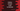# How to convert octal to decimal in python## Python program to convert octal to decimal:

In this post, we will learn how to convert a octal value to decimal. This program will take the octal value as input from the user and convert it to decimal.

Octal number system is a base-8 number system. Each number is represented by the digits 0 to 7.

We can use our own algorithm or we can directly use int() to convert a octal value to decimal.

### Algorithm to convert octal to decimal:

We need to follow the below steps to convert a octal value to decimal:

• Take the octal value as input from the user.
• Multiply each digits of the number with power of 8. The rightmost digit with 8^0, second right digit with 8^1 etc. Also, add all the values calculated.
• The total sum will result the required decimal value.

### Python program:

Below is the complete python program:

``````def octal_to_decimal(n):
decimal = 0
multiplier = 1

while(n):
digit = n % 10
n = int(n/10)
decimal += digit * multiplier
multiplier = multiplier * 8
return decimal

no = int(input('Enter the octal value : '))
print('Decimal value is : {}'.format(octal_to_decimal(no)))``````

Here,

• octaltodecimal method is used to convert a octal value to decimal. It takes one octal value and returns the decimal conversion.
• It uses a while loop that picks the rightmost digit and multiply with power of 8 and adds it to the decimal variable, which is the final decimal value.
• It asks the user to enter a number. It sores the number in the variable no and passes the value to octaltodecimal to convert it to a decimal value.

### Sample output:

If you run this program, it will print the below output:

``````Enter the octal value : 35
Decimal value is : 29``````

### Method 2: Using int():

We can also use int() with the octal number as the first argument and 8 as the second argument to convert the octal value to decimal.

``````no = input('Enter the octal value : ')
print('Decimal value is : {}'.format(int(no, 8)))``````

It will print similar output.